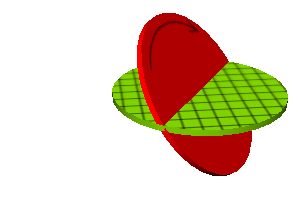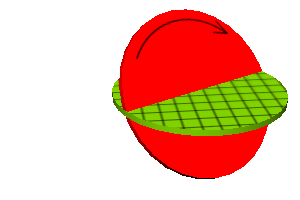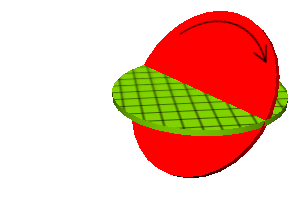[Contents]

A Brief Review of the Concept of Spin
John Denker

## 1  Introduction

Quantum-mechanical spin is one of those things that is so fundamental that it cannot be defined in terms of anything more fundamental. Therefore we are better off asking what it does rather than what it is. (You could say the same thing about energy, or about charge.)

This document is meant to be a terse review of the basic facts – not a tutorial. Most of the facts will be asserted without proof. If you want proofs, or more-detailed explanations, consult any book on quantum mechanics. Reference 1 is a good place to start. Reference 2 has decent coverage of the fundamental principles that underlie atomic physics.

Spin is connected to angular momentum. We are familiar with the idea of spin in connection with the motion of the earth. The total angular momentum of the earth includes the following contributions:

• The spin angular momentum. The earth spins on its axis once per sidereal day.
• The orbital angular momentum. The earth orbits the sun once per year.

The same words apply to a particle such as an electron, neutron, photon, atom, or atomic nucleus. It generally has spin angular momentum plus orbital angular momentum.

The spin angular momentum of the earth can be explained by the macroscopic motion of the particles that make up the earth, as they circulate around the center of the earth, once per sidereal day, in accordance with classical physics ... but this is provably not true for the spin angular momentum of the electron. The spin angular momentum is non-classical and is not associated with the motion of any “stuff” inside the electron. Any attempt to give a classical explanation of what spin is will necessarily fail at this point.

Angular momentum is a conserved quantity.

You may have heard angular momentum described as a vector, aligned with the axis of rotation. Actually, there are numerous reasons why it is better to think of it as a bivector in the plane of rotation, e.g. the equatorial plane of the earth. (This is true for orbital angular momentum and for spin angular momentum as well.) Figure 1 is an example of this, representing the bivector as an oriented patch of area. See reference 3 and references therein for more about bivectors. In any case, it has direction and magnitude. You can make a hands-on demonstration of angular momentum using a bicycle wheel with handles: Because the wheel spins on good bearings, you cannot change the magnitude of the angular momentum by means of the handles, but you can change the direction, and you can very clearly feel the torque required to change the direction.

If we pick a basis, we can expand the angular momentum in terms of components. Conservation of angular momentum implies that each component is separately conserved.

Actually, for reasons that will become apparent shortly, we choose to work with the bivector S, which is the spin angular momentum divided by ℏ. This is simpler than working with the angular momentum bivector directly. Using the usual Cartesian basis, we can write S in terms of components: S = [Sxy, Syz, Szx].

A funny thing about quantum mechanics is that (for any given particle) we cannot measure all three components of S at the same time. There is a Heisenberg-like uncertainty principle at work. If we measure Sxy it messes with Syz and Szx, and similarly for the others. The uncertainty in S is on the order of 1. That is to say, the uncertainty in the angular momentum is on the order of ℏ, which means you will never notice it in classical situations, but it is quite significant for electrons etc. in atoms.

With a little cleverness we can measure the scalar:

 S2 = S·S = Sxy2 + Syz2 + Szx2
(1)

We can measure this combination of components without disturbing any of the components, even though we cannot say the same about any of the separate terms on the RHS of this equation.

When S2=0, as for an alpha particle, we know that S=0 which means all three components of S are necessarily zero. Otherwise, when S2 is nonzero, the best we can do is to measure S2 and one of the components of S. For particles at rest, it is conventional to pick the Sxy component. For massless particles such as photons, it makes more sense to pick the component in the plane transverse to the direction of motion.

Let’s be clear: For any particle, there are three spin quantum numbers, but we can never have good values for more than two of them at a time (unless they are all zero) because there is an uncertainty principle involved. In the following examples, we follow convention and pick S2 and Sxy to be the knowable spin quantum numbers.

For an alpha particle, we have:

 s S2 Sxy 0 0 0
(2)

For an electron, proton, or neutron, S2 is always 0.75. Since it is always the same, we don’t talk about it much, but it is what it is. The “interesting” quantum number is Sxy. The two states of definite spin are:

 s S2 Sxy words 1/2 3/4 +1/2 spin up 1/2 3/4 −1/2 spin down

(3)

The particle can also be in some superposition of those states. In particular, it is easy to put an electron into a superposition state where the expectation value of Sxy is zero. If you measure Sxy you get a 50/50 chance of spin up versus spin down.

For a hydrogen atom, there are four states of definite spin:

 s S2 Sxy 0 0 0 1 2 +1 1 2 0 1 2 −1

(4)

The atom can be in some superposition of those states. There are an infinite number of possibilities, but these four states are a basis that spans the space.

The hydrogen atom is made up of a proton and electron, each of which can be in either of two spin states, so it makes sense that the combination would have four spin states.

For a deuteron (i.e. deuterium nucleus), there are only three states of definite spin:

 s S2 Sxy 1 2 +1 1 2 0 1 2 −1

(5)

Since a deuteron is made of a proton and a neutron, each of which has two spin states, you might think the deuteron would have four spin states. It would, except that the S=0 state is energetically disfavored. The state is never observed. If you tried to prepare such a state it would immediately dissociate into separate particles.

For a copper nucleus under ordinary conditions, there are four spin states:

 s S2 Sxy 3/2 15/4 +3/2 3/2 15/4 +1/2 3/2 15/4 −1/2 3/2 15/4 −3/2

(6)

It turns out that S2 can always be written in the form S2 = s(s+1) for some integer or half-integer s. The s-values are shown in the examples tabulated above. For any such s-value, Sxy takes on values from −s to s inclusive, in steps of 1. That means there are 2s+1 different values for Sxy. We say the multiplicity is 2s+1. You can check that all the examples given above conform to this pattern.

## 2  Terminology

Now, here is something horrible: Both s and Sxy are conventionally called “the” spin. It is quite common for experts in the field to use the word spin twice in a sentence, with two different meanings. For example: “This deuteron is a spin-one particle in the spin-zero state.” It’s clear from context that the meaning is s=1 and Sxy=0 ... but newcomers to the field tend to tear their hair out when they hear this sort of ambiguous language.

By way of analogy, imagine what would happen if the momentum vector p and the energy p·p/2m were both called by the same name. Actually, there was a time in the early history of mechanics when some folks thought p was the vis viva and other folks thought p2 was the vis viva. There were arguments about it, until they gave these things separate names and realized that both were useful.

Constructive suggestion: To get around the ambiguity of the word “spin”, use the symbols. To restate the previous example: “This deuteron has s=1 and Sxy=0.”

Another way of lifting the ambiguity is to specify the s-value by mentioning the multiplicity. For hydrogen we have:

 s S2 Sxy words 0 0 0 singlet 1 2 +1 spin up 1 2 0 triplet spin zero 1 2 −1 spin down

(7)

In this narrow context “spin” refers to Sxy (but in any other context, all bets are off). Here “singlet” is shorthand for “singlet spin zero” which makes sense because singlet means s=0 which implies S=0 which in turn implies Sxy must be zero. Meanwhile “spin up” is shorthand for “triplet spin up” which makes sense because in hydrogen the only state that could possibly be called “spin up” is the triplet spin up state.

As a relatively minor point of terminology, in chemistry and atomic physics, Sxy is commonly denoted ms or even simply m. This is mostly harmless, but conceals the fact that Sxy is just one component of a bivector. Remember that all three components of S are separately conserved; Sxy aka ms is not the only conserved quantity.

## 3  Quantization

Orbital angular momentum is always an integer (in units of ℏ).

The spin angular momentum of a fermion is always an odd half-integer (in units of ℏ). Examples include the electron, proton, neutron, and copper nucleus as tabulated above.

The spin angular momentum of a boson is always an even half-integer, i.e. an integer (in units of ℏ). Some examples include the deuteron and the hydrogen atom as tabulated above.

This means, among other things, that any transfer of angular momentum is always quantized as an integer multiple of ℏ. This spin/spin interactions, spin/orbit interactions, and every other possibility.

Any particular component of the angular momentum (such as ℏ Sxy), if it has a definite value, will be quantized in units if ℏ/2. (Beware: It does not necessarily have any definite value. You can easily prepare a superposition state that involves a superposition of multiple Sxy values.)

The magnitude of the total spin angular momentum ℏ |S| is not quantized. It can take on all sorts of values, including irrational values.

## 4  Non-Alignment

In this section we assume s is nonzero.

It is amusing to compare s to the norm of S, i.e.

|S| =
 S·S

=
 s(s+1)

> s     for nonzero s
(8)

For the rest of this section, we focus attention on a state where Sxy has the largest possible value consistent with a given s, namely Sxy = s. (This is called a stretched state.) We see from equation 8 that for nonzero s, |S| is strictly greater than s. That means that the total angular momentum of the particle is never completely aligned with the xy plane. It doesn’t matter how hard you try to align it; you will never completely succeed. There will always be some Syz and Szx components that you can’t get rid of ... and can’t even measure without messing up Sxy.

 s s(s+1) |S| |S|−s θ 0.0 0.00 0 0 undef 0.5 0.75 0.87 0.37 54.74 1.0 2.00 1.41 0.41 45.00 1.5 3.75 1.94 0.44 39.23 2.0 6.00 2.45 0.45 35.26 2.5 8.75 2.96 0.46 32.31 3.0 12.00 3.46 0.46 30.00 3.5 15.75 3.97 0.47 28.13 4.0 20.00 4.47 0.47 26.57 4.5 24.75 4.97 0.47 25.24 5.0 30.00 5.48 0.48 24.09 50 2550 50.50 0.50 8.05 200 40200 200.50 0.50 4.04 800 640800 800.50 0.50 2.02

In the table above, the next-to-last column shows the difference between |S| and s. Perhaps even more interesting is the angle between the stretched state’s bivector S and the XY plane. The last column of the table gives this angle (in degrees). For particles that have s=1/2 (e.g. electrons and protons), this is a rather large angle, 54.74 degrees.

This tells us that the angular momentum S is always inclined relative to the XY plane. For a stretched state, the the angle of inclination, θ, is given in the table above. (For a non-stretched state, the inclination would be even larger.) In any case, we do not know the azimuthal angle. That is, we are uncertain as to the longitude of the direction that the S bivector is facing. This uncertainty is required by the laws of nature.

This situation is shown in the following figures. The XY plane is shown in green, with a grid representing contours of constant X and contours of constant Y. The spin bivector is shown in red, with an arrow indicating the direction of spin. In all three figures, the angle of inclination is the same, namely 54.74 degrees, as appropriate for a spin-1/2 particle. We are certain as to the value of |S| and the value of the Sxy component, but uncertain as to the other two components of S. For any particular particle, we know the Syz and Szx components are not randomly fluctuating, since that would violate conservation of angular momentum. Those components must have some constant value; we just don’t know what that value is.Figure 1: Spin – Off Axis – Some AzimuthFigure 2: Spin – Off Axis – Another AzimuthFigure 3: Spin – Off Axis – Yet Another Azimuth

Let us now consider the limit of large s. This is called the classical limit. As you can see in the table above – and can easily verify algebraically – the stretched state behaves as follows:

• The algebraic difference |S| − s converges to 0.5.
• The ratio |S|/s converges to 1.
• The angle between the stretched state and the XY plane converges to zero. It converges rather slowly, but it does converge.

The bottom line is as we expected: In the classical limit, you will not notice the non-alignment.

## 5  Fine Structure of Spectral Lines

For any charged particle (and for some uncharged particles, such as the neutron), spin is associated with a built-in magnetic moment. Each electron is therefore a little bar magnet. Indeed, an ordinary macroscopic bar magnet is magnetic only to the extent that it contains a lot of unpaired electrons all pointing the same way. That is, none of the magnetism of an ordinary bar magnet comes from orbital angular momentum; all of it comes from spin angular momentum.

The so-called “fine structure” of an atomic spectrum includes a number of terms, including:

• relativistic terms that have got nothing to do with spin.
• spin-orbit couplings
• various other things.

And on top of the so-called fine structure, there is also hyperfine structure.

We note that the Pauli exclusion principle depends in part on the spin of the particles. This principle, applied to the electrons, helps determine the gross structure of the atomic wavefunction. This is not considered part of the fine structure. We also note that to leading order, the electron-electron repulsion and electron-nucleus attraction are considered part of the gross structure of the spectrum, not the fine structure.

We now turn to the fine structure. In this category, the main spin-related piece is the spin-orbit coupling. This can be understood as a purely magnetic interaction involving the orbital motion of one electron (which can be considered an electromagnet) interacting with the spin of another electron (which can be considered a bar magnet).

The hyperfine interaction is even easier to understand: It is the magnetic interaction between the spin of the electron and the spin of the nucleus.

## 6  References

1.
Feynman, Leighton and Sands, The Feynman Lectures on Physics Volume III (Addison-Wesley, Reading, MA, 1965).

2.
Gordon Baym, Lectures on Quantum Mechanics (1969).

3.
John Denker, “Introduction to Clifford Algebra” www.av8n.com/physics/clifford-intro.htm

4.
Wikipedia article, “Spin (physics)” http://en.wikipedia.org/wiki/Spin_(physics)

5.
Wikipedia article, “Fine Structure” http://en.wikipedia.org/wiki/Fine_structure
[Contents]